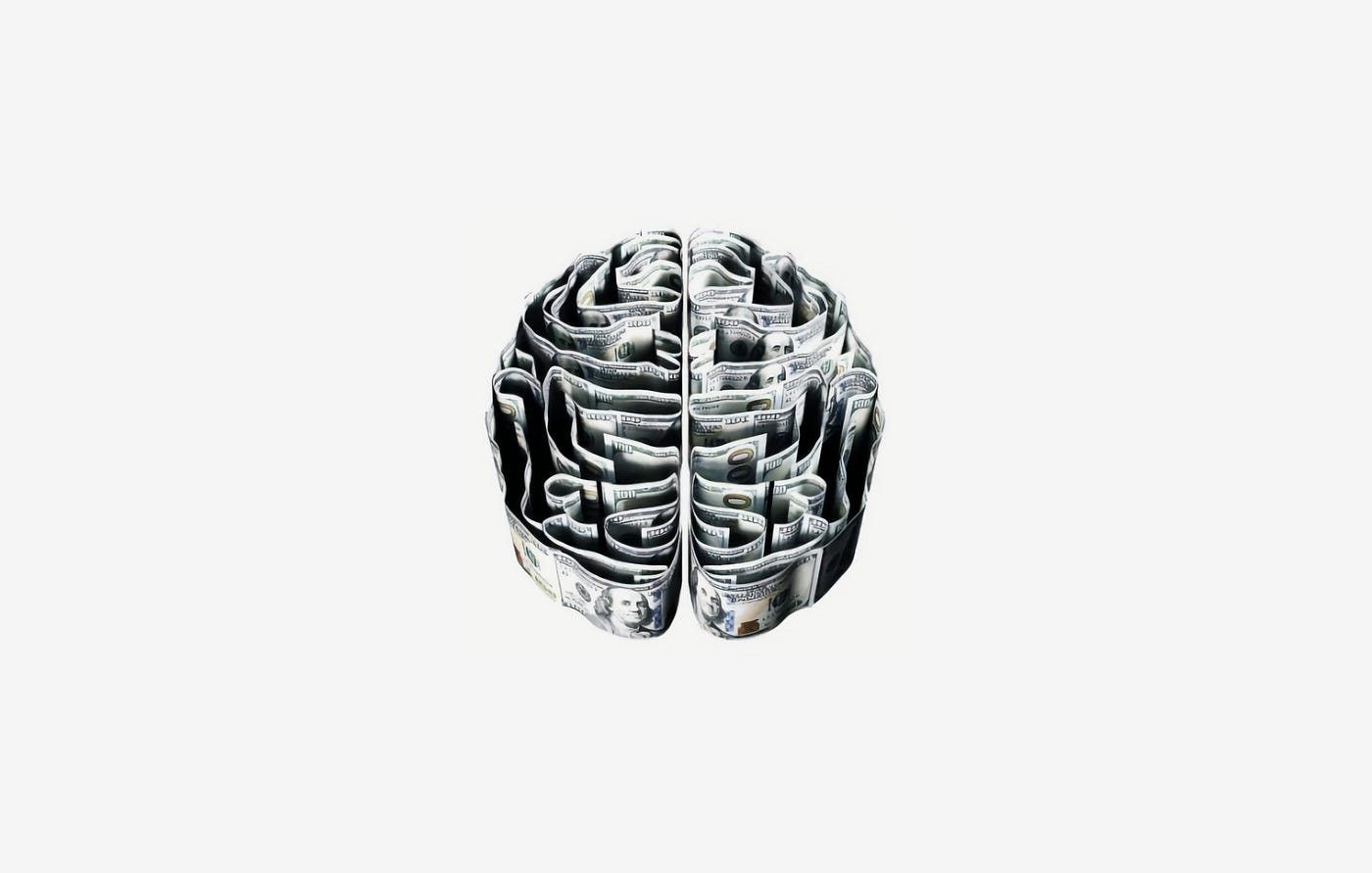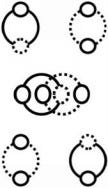# Knowledge: As a Service

Where does knowledge come from?Photo by Morgan Housel on Unsplash

To differentiate (separate) (join) X and Y you need an uber-simple circle.

This is because, from a circle’s point of view, there is no such thing as X and Y. Even though, paradoxically, there is a mandatory circle between X and Y (any X and-or Y) (every X and-or Y).

Meaning, technically, and technologically, X and Y is 0 and 1, circumference and diameter, literally and figuratively.X and Y (0 and 1) (Circumference and Diameter)

## Pi Controls Reality (Knowledge in General)

Meaning, pi controls reality. Explaining why the brain is divided into two.

Because you need circumference for diameter, you need left for right, any X for Y. (X for X) (X for X’). (D for NA).

This means 50–50 is the constant and the norm (for everything).

## You Have to Use The Diagram (If You Want to Understand What is Really Going On)

Therefore, all symbolic representations (language, numbers, diagrams) (units in any discipline) (units in any system) articulate, and, thus conserve, an uber-simple circle.

Thus, everything a ‘brain’ processes is turned into the diagram above (and below).

Meaning you cannot have up without down, left without right, any X without an alternate-X. Explaining negation and duplication. Information and knowledge in general.

## Nature Uses The Human (And Everything Else) As A Proxy (For an Uber-Simple Circle)

That is, no matter what we’re saying (and-or seeing) an uber-simple circle has control (it sees for us) (it speaks through us). (See, also, https://medium.com/the-circular-theory/kaas-knowledge-as-a-service-58dea9e8e295)

Conservation of the Circle is the core, and, therefore, the only dynamic in Nature. Explaining knowledge, information, and reality, then, in general. (If X, then Y, has its basis within a circle because if circumference, then diameter, explaining why the number ‘two’ is the core assumption that is used for everything.)